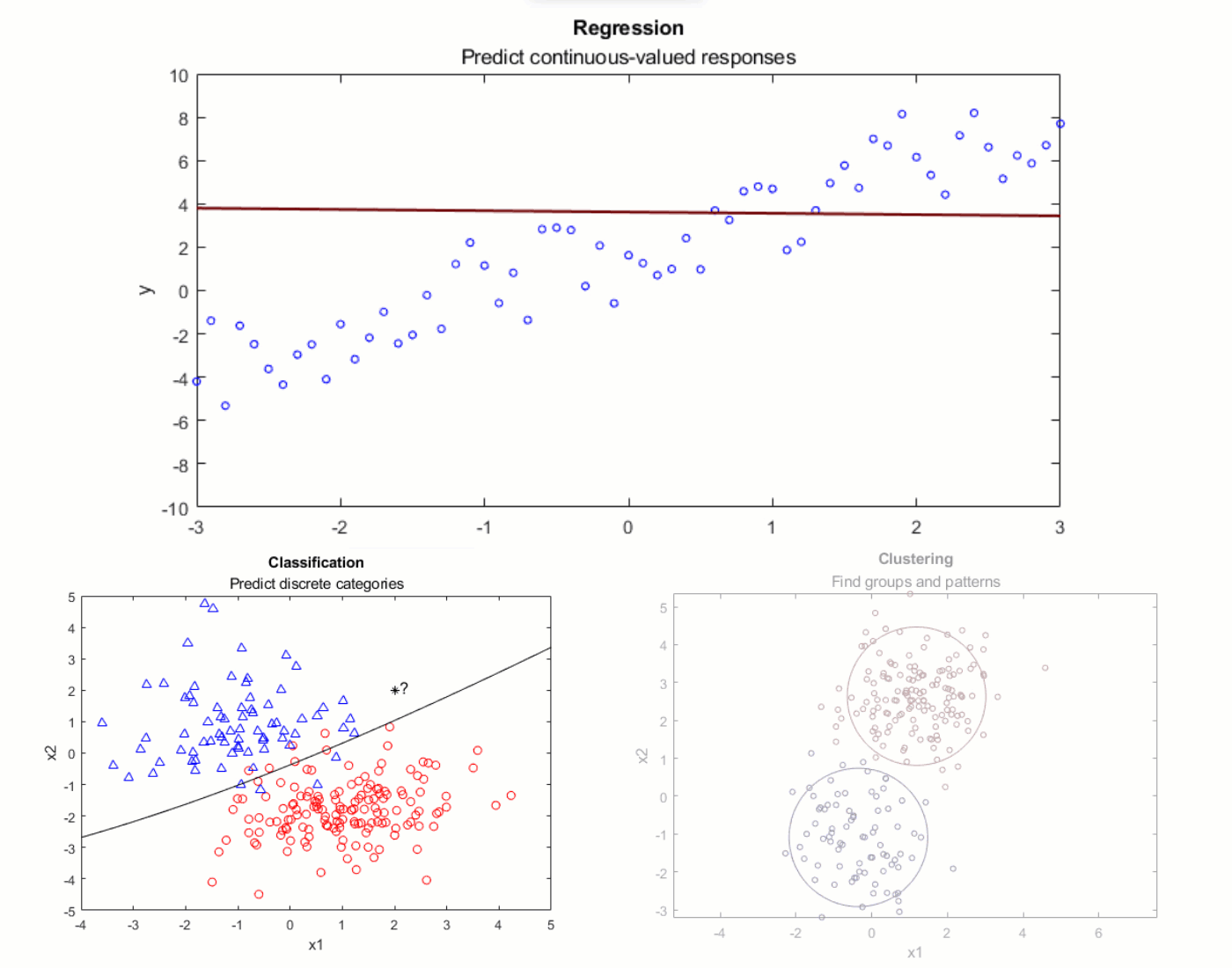## Machine Learning for Regression

version 1.1.2 (3.72 MB) by
Interactive module that introduces typical workflow, setup, and considerations involved in solving regression problems with machine learning

Updated 26 Aug 2022

From GitHub

# Machine Learning for Regressionor Open in MATLAB Online

Curriculum Module
Created with R2021a. Compatible with R2021a and later releases.

## Description

This package contains several live scripts and supporting files that teach the basics of machine learning for regression. The materials are designed to be flexible and can be easily modified to accommodate a variety of teaching and learning methods. These include a brief background, interactive illustrations, tasks, reflection questions, a real-world application in electricity load forecasting, and guided exercises for the different concepts explored. The module can be used to provide a light introduction to the terminology and concepts in machine learning, centered around regression. The overarching goal is to familiarize students with the typical workflow, setup, and considerations involved in solving regression problems with machine learning.

The instructions inside the live scripts will guide you through the activities and exercises. Get started with each live script by running it one section at a time. To stop running the script or a section midway (for example, when an animation is in progress), use the Stop button in the RUN section of the Live Editor tab in the MATLAB toolstrip.## Suggested Prework

MATLAB Onramp—a free two-hour introductory tutorial to learn the essentials of MATLAB®. Additional programming skills (see MATLAB Fundamentals) are beneficial, but not assumed in the tasks and instructions.
Regression Basics—a curriculum module to cover the fundamentals of regression analyis.

No prior exposure to the subject of machine learning is assumed.

## Details

`machineLearningIntro.mlx` Open in MATLAB Online An interactive lesson that introduces some key concepts in machine learning, along with a few regression models. It contains many independent introductory sections that are easy to edit.

Learning Goals

• State the difference between regression, classification, and clustering problems.
• Outline the common steps involved in applying machine learning techniques.
• Define feature engineering and feature extraction.
• Formulate regression as a machine learning problem.
• Identify and use the different machine learning models commonly used for regression.
• Explain overfitting and underfitting in machine learning, and identify at least two ways of tackling these problems.

`loadForecastRegression.mlx`, `loadForecastRegression_soln.mlx` Open in MATLAB Online
Students are guided through the steps to apply machine learning for electricity load forecasting using real-world data. This script can be used in two different modes: controls-only or with complete code.

Learning Goals

• Apply the steps in the machine learning workflow to solve a practical problem in time series forecasting.
• Formulate the time series forecasting problem as a machine learning problem by engineering appropriate features.
• Validate and compare different types of regression models.
• Test and evaluate the trained model to make predictions.

`electricityLoadDataML.mlx` Open in MATLAB Online
A supplementary script to download the external electricity load data from New York ISO for use in `loadForecastRegression.mlx`. This script contains the code for downloading, organizing, formatting, and cleaning up the raw data.

`FE1_programmaticML.mlx`, `FE2_loadForecastDL.mlx` Open in MATLAB Online
These two scripts contain ideas to expand on the practical problem presented in `loadForecastRegression.mlx`. Working through the suggestions requires some independent exploration and active learning. `FE1_programmaticML.mlx` encourages students to write their own machine learning algorithm, and `FE2_loadForecastDL.mlx` begins to explore deep learning for load forecasting.

## Products

MATLAB, Statistics and Machine Learning Toolbox™

The license for this module is available in the LICENSE.TXT file in this GitHub repository.

## Support

Have any questions or feedback? Contact the MathWorks online teaching team.

Copyright 2021 The MathWorks, Inc.

### Cite As

Emma Smith Zbarsky (2022). Machine Learning for Regression (https://github.com/MathWorks-Teaching-Resources/Machine-Learning-for-Regression/releases/tag/v1.1.2), GitHub. Retrieved .

##### MATLAB Release Compatibility
Created with R2021a
Compatible with R2021a and later releases
##### Platform Compatibility
Windows macOS Linux
##### Communities
More Files in the  Distance Learning Community

### Community Treasure Hunt

Find the treasures in MATLAB Central and discover how the community can help you!

Start Hunting!
To view or report issues in this GitHub add-on, visit the GitHub Repository.
To view or report issues in this GitHub add-on, visit the GitHub Repository.